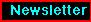HEASARC support for unencrypted FTP access will be ending on September 20, 2019. Please see this notice for details.Off-axis Point Spread Function (PSF) for the ROSAT HRI:

February 1994

The on-axis HRI PSF can be described with two gaussians plus an exponential (due to the HRI halo):

PSF = A1*exp(-0.5*(r/s1)**2) + A2*exp(-0.5*(r/s2)**2) + A3*exp(-r/s3)

with

s1 = 2.18, A1 = 0.96, s2 = 4.04 , A2 = 0.18, s3 = 31.7, A3 = 0.00090

where r, s1, s2, and s3 are in arc seconds. The on-axis PSF was determined from a long observation of HZ43 that produced 100,000 net counts. Analysis of a sample of point sources observed on-axis (mostly stars), shows that the HRI PSF varies from observation to observation (due to residual errors in the aspect solution). Fitting the above function form of the PSF to this sample shows that s1 varies from 2.0 to 3.0 arc seconds, and s2 varies from 3.2 to 4.6 arc seconds. When possible, users should use the image of a known point source within 5" of their target as a template for the HRI PSF.

We recently derived a parametric representation of the azimuthally averaged off-axis HRI PSF that is a simple extension of the above expression. To determine the off-axis PSF, we generated surface brightness profiles for eight observations of HZ43, spanning a range of 2" to 16" off-axis. The peak surface brightness was used as the origin, since this origin generates a monotonically decreasing profile. The set of eight HZ43 surface-brightness profiles were first fit to the on-axis PSF, treating the normalization and width of the two gaussians as free parameters (the photon statistics in these data were insufficient to fit the exponential term). We found that the best -fit values of the smaller gaussian were essentially independent of the angle off-axis. We then refit the set of eight profiles treating only the width of the broader gaussian as a free parameter. The best fit values of s2 are well parameterized by a cubic polynomial in the off-axis angle, and are given by

s2 = 3.3 + 0.019*theta -0.016*theta**2 + 0.0044*theta**3

where theta is the angle off-axis in arc minutes. Substituting this expression into the on-axis HRI PSF gives the general off-axis representation. Note, however, that we do not force this expression to reproduce the on-axis value given above. The fact that this expression gives s2 = 3.3 for theta=0, compared to the on-axis value of 4.0 given above, reflects the variation in aspect quality from observation to observation mentioned above.

Users should note that point sources observed off-axis exhibit significant amounts of nonazimuthal symmetry. The above expression is useful for determining scattering corrections and for use in detect algorithms, but should not be used for image deconvolution. There are a large number of sequences in the PROS calibration directory xrcal\$ containing several objects observed at different angles off-axis. The actual HRI data should be used for any two-dimensional image deconvolution.

The information given above is also available within IRAF/PROS (type help prfroshri -- note a typo in the help file: A4 should be s3) and in the report The ROSAT High Resolution Imager (HRI) by L.P. David, et al. (1993), which is available from the anonymous ftp site legacy.gsfc.nasa.gov and was distributed with the Solicitation for AO5 Observing Proposals.Select another edition of the ROSAT Newsletter

Curator: Michael Arida (SP Sys); arida@milkyway.gsfc.nasa.gov
HEASARC Guest Observer Facility